# Java泛型详解,通俗易懂只需5分钟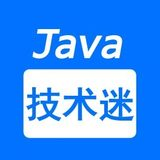Java技术迷 |184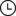2022-01-13 16:49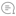0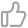00Java |

01

``public class Main {    public static void main(String[] args) {        List list = new ArrayList();        list.add(1);        list.add(2);        list.add(3);        dealList(list);    }    public static void dealList(List list) {        int result = 0;        for (Object obj : list) {            int num = (int) obj;            result += num;        }        System.out.println(result);    }}``

``public static void main(String[] args) {    List list = new ArrayList();    list.add(1);    list.add(2);    list.add(3);    list.add("4");    dealList(list);}``

``Exception in thread "main" java.lang.ClassCastException: java.lang.String cannot be cast to java.lang.Integer``

02

``@Data@AllArgsConstructor@NoArgsConstructorpublic class Genericity {    private String str1;    private String str2;}``

``public static void main(String[] args) {    Genericity genericity = new Genericity("1","1");       String str1 = genericity.getStr1();    String str2 = genericity.getStr2();    Integer num1 = Integer.valueOf(str1);    Integer num2 = Integer.valueOf(str2);    int result = num1 + num2;    System.out.println(result);}``

``@Data@AllArgsConstructor@NoArgsConstructorpublic class Genericity {    private Integer str1;    private Integer str2;}``

``@Data@AllArgsConstructor@NoArgsConstructorpublic class Genericity<T> {    private T t1;    private T t2;}``

``public static void main(String[] args) {    Genericity<Integer> genericity = new Genericity<>(1, 1);    Integer t1 = genericity.getT1();    Integer t2 = genericity.getT2();    int result = t1 + t2;    System.out.println(result);}``

``@Data@AllArgsConstructor@NoArgsConstructorpublic class Genericity<T,U> {    private T t1;    private U t2;}``

``public static void main(String[] args) {    Genericity<Integer,String> genericity = new Genericity<>(1, "1");    Integer t1 = genericity.getT1();    String t2 = genericity.getT2();}``

03

``public static <T> void method(T t) {}``

``public static void main(String[] args) {    Genericity.<Integer>method(1);}``

``public static void main(String[] args) {    Genericity.method(1);}``

``public static <T> T method(T t) {    return t;}``

``public static <T,U> T method(T t,U u) {    return t;}``

04

``public static <T> T min(T[] t) {    T minimum = t;    for (int i = 0; i < t.length; i++) {        if (minimum.compareTo(t[i]) > 0) {            minimum = t[i];        }    }    return minimum;}``

``public static <T extends Comparable> T min(T[] t) {    T minimum = t;    for (int i = 0; i < t.length; i++) {        if (minimum.compareTo(t[i]) > 0) {            minimum = t[i];        }    }    return minimum;}``

``public static <T extends Comparable & Serializable> T min(T[] t) {    ......}``

05

``public static void main(String[] args) throws Exception {    List<Integer> list = new ArrayList<>();    list.add(1);    Method addMethod = list.getClass().getDeclaredMethod("add",Object.class);    addMethod.invoke(list,"2");    addMethod.invoke(list,true);    addMethod.invoke(list,3.2f);    System.out.println(list);}``

``[1, 2, true, 3.2]``

``public static <T extends Comparable> T min(T[] t) {    ......}``

``public static Comparable min(Comparable t) {    ......}``

06

``Genericity<? extends Person>``

``Genericity<? super Person>``

``Genericity<?>``

``public static boolean isNull(Genericity<?> genericity){    return genericity.getContent();}``
`本文作者：汪伟俊 为Java技术迷专栏作者 投稿，未经允许请勿转载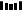往期推荐1、致歉！抖音Semi Design承认参考阿里Ant Design2、对比7种分布式事务方案，还是偏爱阿里开源的Seata，真香！3、Redis存储结构体信息，选hash还是string？4、扫盲 docker 常用命令5、最全分布式Session解决方案6、21 款 yyds 的 IDEA插件7、真香！用 IDEA 神器看源码，效率真高！点分享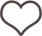点收藏点点赞点在看`00

暂无评论~~
Ctrl+Enter